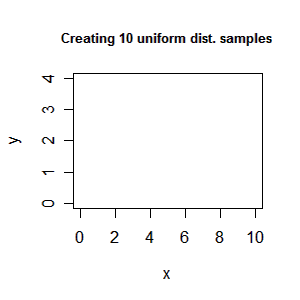# Creating an animation (gif/mpeg) in R using intermediate files

I recent had a discussion with Søren about how one could create an animation of some plots in R. After searching the mail-list it seems that the best way to do it is using ImageMagick which is a free set of tools to create, edit, and compose bitmap images. To use the following guide you must install ImageMagick.

Creating an animated gif

We create an animated gif file in two steps. First, we save all the plots used in the animation as png files (vector file format) and second, we merge them into a gif animation.

Lets try a simple example:

> x<-1:10
> y<-runif(10,1.5,2.5)
> xlim<-c(0,10)
> ylim<-c(0,4)
> png(file="plot%02d.png", bg="transparent")
> plot(x, y, type="n", xlim=xlim, ylim=ylim)
> title("Create 10 uniform distributed samples")
> for (i in 1:10) plot(x[i], y[i], axes=F, xlab="", ylab="", xlim=xlim, ylim=ylim)
> dev.off()

We have now created intermediate files plot01.png to plot11.png. Note

1. Plot plot01.png only contains the axes and titles of the plot
2. The rest of the plots only contain the single point we want to add to the animation. That is we want to put the point on top of the frames (keeping the old ones).

Finally we merge the files

 > system('convert -delay 10 -dispose None plot*.png -loop 0 plot.gif', invisible = F, wait=T)The -despose option ensures that the images are put on top of each other (therefore the background must be transparent). Option -despose background can be used if you don’t use transparent images. Many other options can be added see the ImageMagick documentation of convert for more details. It might be usefull to reduce the file size of the gif (takes longer than creating plot.gif)

> system('convert -coalesce -layers OptimizeFrame plot.gif plot1.gif', invisible = F, wait=T)

Creating an mpeg

Unfortunately, gifs cannot be embedded into a pdf file so if you have a pdf presentation you must create an mpeg movie instead. The easiest way is just converting your gif into a mpeg; however, since the convert program in ImageMagick is not so fund of transparent backgrounds in gifs when converting to an mpeg you must fix this first

> png(file="background.png", bg="white")
> plot(x,y,type="n",xlim=xlim,ylim=ylim)
> dev.off()
> system('convert  background.png  null: ( plot.gif -coalesce ) -gravity Center -layers Composite -layers Optimize plot2.gif',invisible = F,wait=T)
> system('convert plot2.gif plot.mpeg',invisible = F,wait=T)

First we create a no transparent background and merge it to all the frames and afterwards we convert the gif to an mpeg which fills much more 🙁 You may use option -quality 1 to reduce file size.